# Propositional Logic

 This is a very simple semi-formal specification of the version of propositional logic called PS by Hunter in [Hunter71]. I call the specification semi-formal because I am not supplying a definition of the metalanguage in which it is written. The system is a classical propositional logic with two connectives (not and implies) presented as a "Hilbert style" axiom system in which there is just one inference rule, modus ponens, and three axiom schemata.

##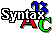::= (any single letter as a propositional variable) |(negation) |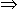(implication)

##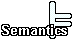An interpretation is a map assigning to each propositional variable one of the two truth values true and false.

The truth value of a formula f under an interpretation i is val i f, where val is a (higher order) function taking two successive arguments, an interpretation i and a propositional formula f. val is defined as follows (function application is shown as juxtaposition, brackets are not necessary for simple arguments):

 val i (f) = if (val i f) = true then false else true val i (f1f2) = if (val i f1) = false then true else (val i f2)

 A formula is tautological (and hence true) if it is true under every interpretation and contradictory (and hence false) if it is false under every interpretation.

##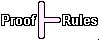There is just one inference rule, modus ponens, and three axiom schemata, as follows.

Modus Ponens
 From two theorems, one of the form:A and the other of the form:AB where A and B are arbitrary formulae, the conclusion:B may be derived.

Axiom Schemata
All instances of the following three schemata are theorems:A(BA)((A(BC))((AB)(AC)))((AB)(BA))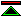©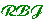created 1997-10-18 modified 1997-10-19